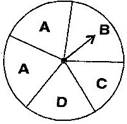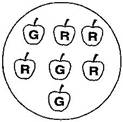# Class 8 NCERT Solutions – Chapter 5 Data Handling – Exercise 5.3

• Last Updated : 05 Nov, 2020

### Question 1. List the outcomes you can see in these experiments.

(a) Spinning a wheel (b) Tossing two coins togetherHey! Looking for some great resources suitable for young ones? You've come to the right place. Check out our self-paced courses designed for students of grades I-XII

Start with topics like Python, HTML, ML, and learn to make some games and apps all with the help of our expertly designed content! So students worry no more, because GeeksforGeeks School is now here!

Solution:

(a) Since, there are four letters A, B, C and D in a spinning wheel therefore there are 4 possible outcomes.

(b) When two coins are tossed together, there are four possible outcomes HH, TT, HT and TH.

### Question 2. When a die is thrown, list the outcomes of an event of getting

(i) (a) a prime number (b) not a prime number.

(ii) (a) a number greater than 5 (b) a number not greater than 5.

Solution:

(i) (a) Outcomes of event of getting a event with prime number are 2, 3 and 5.

(b) Outcomes of event of not getting a event with prime number are 1, 4 and 6.

(ii) (a) Outcomes of event of getting a event with number greater than 5 is 6.

(b) Outcomes of event of not getting a event with number greater than 5 are 1, 2, 3, 4 and 5.

### Question 3. Find the

(a) Probability of the pointer stopping on D in (Question 1-(a))?

(b) Probability of getting an ace from a well shuffled deck of 52 playing cards?

(c) Probability of getting a red apple. (See figure below)Solution:

(a) In a spinning wheel, there are five pointers A, A, B, C and D.

Since there are five outcomes from which the Pointer stops at D. So D is the outcome.

Therefore, the probability of the pointer stop on D =1/5

(b) There are 4 aces in a deck of 52 playing cards(one from each suit i.e. diamond, club , heart and spade).

Since, there are four events of getting an ace. Therefore, probability of getting an ace = 4/52 = 1/13

(c) Total number of apples are 7, out of which number of red and green are 4 and 3 respectively.

Probability of getting a red apple = 4/7

### Question 4. Numbers 1 to 10 are written on ten separate slips (one number on one slip), kept in a box and mixed well. One slip is chosen from the box without looking into it. What is the probability of

(i) getting a number 6?

(ii) getting a number less than 6?

(iii) getting a number greater than 6?

(iv) getting a 1-digit number?

Solution:

Given, there are 10 separate slips in the box.

(i) Number 6 is written only on 1 slip.

Therefore, probability of getting a number 6 is = 1/10

(ii) Numbers less than 6 are 1, 2, 3, 4 and 5 which are 5. Since there are 5 outcomes.

Therefore, probability of getting a number less 6 is = 5/10 = 1/2

(iii) Number greater than 6 out of 10 are 7, 8, 9, 10. Since there are 4 possible outcomes.

Therefore, probability of getting a number greater than 6 is = 4/10 = 2/5

(iv) Since one-digit numbers are 1, 2, 3, 4, 5, 6, 7, 8, 9 out of 10.

Therefore, probability of getting a 1-digit number is = 9/10

### Question 5. If you have a spinning wheel with 3 green sectors, 1 blue sector and 1 red sector, what is the probability of getting a green sector? What is the probability of getting a non-blue sector?

Solution:

A total of 5 sectors are present(3 green + 1 blue + 1 red).

Out of the 5 sectors, 3 sectors are green Therefore, the probability of getting a green sector = 3/5

Out of the 5 sectors, 1 sector is blue. Therefore, Non-blue sectors=5 – 1 = 4 sectors

Therefore, probability of getting a non-blue sector is = 4/5

### Question 6. Find the probabilities of the events given in Question 2.

Solution:

When a die is thrown, there are total six possible outcomes, i.e., 1, 2, 3, 4, 5 and 6.

(i) (a) 2, 3 and 5 are the prime numbers. So there are 3 outcomes out of 6.

Therefore, probability of getting a prime number is = 3/6 =1/2

(b) 1, 4 and 6 are not the prime numbers. So there are 3 outcomes out of 6.

Therefore probability of getting a prime number is = 3/6 = 1/2

(ii) (c) Only 6 is greater than 5.

Since there is 1 outcome out of 6 possible outcomes.

Therefore, probability of getting a number greater than 5 is = 1/6

(d) Number which are not greater than 5 are 1, 2, 3, 4 and 5. Since there are 5 outcomes out of 6.

Therefore, probability of not getting a number greater than 5 is = 5/6

My Personal Notes arrow_drop_up